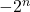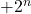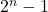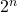# Programming Techniques: Bit hackery

Sean Eron Anderson (Stanford CS graphics lab)’s bit twiddling pages often shows a bunch of neat bit tricks, but it’s more like a recipe book than a unified way to summarize the common concepts behind them. Here’s my attempt. This page will get updated as I got the time and more useful insights collected.

## Concept: Two ways to get two’s complement

This is the basics of most bit hacks below. Sometimes the definition itself is a bit trick on its own.

\begin{align}
\overline{-x}+1=x \\
-x = \overline{x}+1
\end{align}

\begin{align}
\overline{x} = -x-1 \\
\end{align}

the above reads: if you flip the bits, you are getting the negative of it subtracted by 1. e.g. ~4 = -5, ~5 = -6, …, ~(-5) = 4, ~(-4) = 3, …

\begin{align}
x = \overline{-x-1} \\
\end{align}

the above reads: any number can be represented by its negative minus -1, then bit-flipped.

\begin{align}
\overline{-x} = x-1 \\
-x = \overline{x-1}
\end{align}

reads: to flip sign, subtract one first then flip bits

So it means to change signs, you can choose to subtract one first then flip bits or flip bits first then add one.

-x=\overline{x-1} = \overline{x}+1

Let’s try it with 2 instead of 1:

\overline{x-2} = \overline{(x-1)-1} = \overline{x-1}+1 = (\overline{x}+1)+1 = \overline{x}+2

You can generalize it to an arbitrary number by subtracting -1 more under the bar on the left hand side and you will get +1 more on the right hand side. Every extra -1 under the bar (bit flips) shows up as +1 outside the bar (bit flips).

\overline{x-3} = \overline{(x-2)-1} = \overline{x-2}+1 = (\overline{x}+2)+1 = \overline{x}+3

This matches the observation that complement schemes (one’s or two’s) both have increasing magnitude move in opposite directions for positive and negative numbers. Look at this table:

This rule can also read as: magnitude offsets goes in opposite directions

\begin{align}
-x+(n-1)=\overline{x-n} = \overline{x}+n
\end{align}

Note that this is NOT distributing bit-flip to two addition/subtraction despite it resembles it with an important distinction that the sign of n changed without turning into (n-1). If it were to distribute, you’ll get (n-2) on the left-hand-side instead of the (n-1) term because the -1 would have been counted twice under distribution.

Bit flips simply doesn’t distribute over the 4 basic (algebraic field) operations. The two’s complement offset is done once and only once when you change the overall representation no matter how many components you break it down into. It’s merely done to shift over the -0 in one’s complement so there’s an extra space for an extra negative numberwhich its positive counterpartis not representable without starting a new digit.

Note to self: the INT_MIN is just the sign bit of ‘1’ followed by all zeros after.

## Concept: Top bit holds the sign

Sounds simple, but if you keep in mind that (x<0) is really asking to see if the top bit is 1, you can check if two numbers has opposite signs without bit shifting it down by simply XOR-ing them (anything below the top bit are ignored) and use (x^y)<0 to check for the resulting top bit is 1, which signals that the sign bits are different.

## Concept: Sign extensions (the top/sign bit gets drag-copied when right shifted)

When you right shift (in signed integers), the top (sign) bit gets drag-copied (sign extended) by the number of bits you right shifted. (Obviously for signed integers, right shifts are zero-filled)

Can exploit this to

• drag the top (sign) bit all the way down to the bottom (so you either get all 1s or 0s) to provide a conditional mask based on the sign (see below)
1??????? \gg 7 \textnormal{ (i.e. type bit width - 1)} = 11111111_2 = -1_{10} \\
0??????? \gg 7 \textnormal{ (i.e. type bit width - 1)} = 00000000_2 = +0_{10} \\

Signed extensions also means a negative number will stay negative and a positive number will stay positive if you right shift

Sign extension behavior is not guaranteed by 1987 ANSI C, but it’s standard on pretty much anything more modern than that. Just make sure anything that uses this behavior are inlined (so the implementation can be easily swapped out), well documented/commented, and platform checks/switches are in place, and there’s a way to quickly check with the slower but platform independent implementation.

## Concept: Getting a bit mask of a 1s (if true) and all 0s (if false)

The ability to convert a logic evaluation (condition) that gives

\begin{align*}
00000001_2 &= +1_{10} & \mathrm{(true)}\\
00000000_2 &= +0_{10} & \mathrm{(false)}
\end{align*}

into a conditional mask that gives

\begin{align*}
11111111_2 &= -1_{10} & \mathrm{(true)}\\
00000000_2 &= +0_{10} & \mathrm{(false)}
\end{align*}

is the basis of many branchless ‘drop/keep this if that’ operations.

This can also be achieved by

• putting a minus sign in front, such as -(cond) that will convert a (+1, 0) into (-1, 0), or
• more efficiently exploiting sign extensions by dragging the top bit to the bottom (by right shifting by the type’s bit length-1)
• computing absolute using the two’s complement’s definition of flip all bits and add 1: drag out a mask that shows that sign, which happens to be a do nothing if all 0s and flip all bits if all 1s in an xor, while the mask of all 1s, which is -1, when subtracted, becomes +1 needed to finish the two’s complement (and that’s subtract by 0 for already positive value).

## Concept:sets all binary digits below it to a stream of 1s

When you count binary numbers up, you must exhaust all the lower digits by filling them with all 1s before you get to advance to (set) a new digit on the left of them. For example,

\begin{align*}
1000_2 &= +8_{10}\\
0111_2 &= +7_{10}
\end{align*}

This can be exploited to create bit-masks that preserves all digits on the left of the first ‘1’ seen from the right (LSB), ‘0’ at that lowest (LSB) set bit (aka ‘1’), and all ‘1’s below it.

\begin{align*}
0110,1000_2 &= +104_{10}\\
0110,0111_2 &= +103_{10}
\end{align*}

Binary digits are are the (1 or 0) coefficients of a linear combination of powers of 2. Having a loner ‘1’ (aka everything else is 0) means the number is a power of 2.

Being the lone ‘1’ bit in the number means every bit above it must be zero. Any ‘1’s above the right-most ‘1’ means it’s not the loner, hence not a power of 2.

If you subtract 1 from the power-of-2 number, only all bits below (not including) the line ‘1’ bit becomes 1, and that ‘1’ bit position become zero, and as mentioned before, all bits above it are 0s by definition since the ‘1’ we are working on is a loner.

Since the digits inandare mutually exclusive (see example below)

\begin{align*}
0000,1000_2 &= +8_{10} = 2^3 \\
0000,0111_2 &= +7_{10} = 2^3 -1
\end{align*}

we can be sure that if we AND them we must get 0 (because one of them has to be zero) and if we XOR them we must get 1. But which one to use?

\begin{align*}
0000,1000_2 &= +8_{10} &=& 2^3 \\
0000,0111_2 &= +7_{10} &=& 2^3 -1 \\
0000,1111_2 &= +15_{10} &=& 2^3 \textnormal{ or } (2^3 -1)\\
0000,1111_2 &= +15_{10} &=& 2^3 \textnormal{ xor } (2^3 -1)\\
0000,0000_2 &= +0_{10} &=& 2^3 \textnormal{ and } (2^3 -1)\\
\end{align*}

Let’s also check for the non-power of two case

\begin{align*}
0110,1000_2 &= +104_{10}\\
0110,0111_2 &= +103_{10}\\
0110,1111_2 &= +111_{10} &=& 104_{10} \textnormal{ or } 103_{10}\\
0000,1111_2 &= +15_{10} &=& 104_{10} \textnormal{ xor } 103_{10}\\
0110,0000_2 &= +96_{10} &=& 104_{10} \textnormal{ and } 103_{10}\\
\end{align*}

The xor approach does not work because the upper bits are invariant, so we cannot detect the presence of the upper set bits (upper ‘1’s). It unconditionally gives the same bit pattern (mask) marking the lowest first set bit and everything below it 1s and 0s for everything else above it. Which can be exploited to simplify counting the consecutive trailing zeros (from the right) by turning it into counting the contiguous 1s in this invariant pattern, or add 1 to it and binary search the position of the set bit and subtract 1 because the said bit was made into the invariant (xor) pattern as well so +1 move onto the next upper binary digit.

The or approach detects the presence of the upper set bits but it’s a pain to mask out the invariant lower 1s, which curiously you can do by XOR-ing with the invariant pattern generated by ]latex]2^n \textnormal{ xor } 2^n-1[/latex] or you can do AND-NOT-ing

\begin{align*}
0110,1000_2 &= +104_{10}\\
0110,0111_2 &= +103_{10}\\
0110,1111_2 &= +111_{10} &=& 104_{10} \textnormal{ or } 103_{10}\\
0000,1111_2 &= +15_{10} &=& 104_{10} \textnormal{ xor } 103_{10}\\
1111,0000_2 &= +240_{10} &=& \overline{104_{10} \textnormal{ xor } 103_{10}}\\
0110,0000_2 &= +96_{10} &=& (104_{10} \textnormal{ or } 103_{10}) \textnormal{ and } \overline{(104_{10} \textnormal{ xor } 103_{10})}\\
0110,0000_2 &= +96_{10} &=& (104_{10} \textnormal{ or } 103_{10}) \textnormal{ xor } (104_{10} \textnormal{ xor } 103_{10})\\
0110,0000_2 &= +96_{10} &=& 104_{10} \textnormal{ and } 103_{10}\\
\end{align*}

Which and happens to already does the job by keeping the top bits (which non-zero value detects their presence) yet unconditionally clear the lowest set bit and everything below it.

The gut of is x & (x-1) maneuver is that it clears the bit from the lowest set bit and everything down below

clearLowestSetBitAndEverythingBelow(x): x & (x-1)

This is used by Brian W. Kernighan to count number of set bits by knocking them one off at a time starting from below. Of course the worst case scenario is when the 1s are so dense that the algorithm must go through every bit without jumping past the zeros.

So the solution is

isPowerOfTwo(x): clearLowestSetBitAndEverythingBelow(x)==0
isPowerOfTwo(x): (x & (x-1))==0

However a special case escaped us, which is x=0. 0x0000 & 0xFFFF is 0, but 0 isn’t a power of 2 unless you consider the minus infinity power which is the territory of floating point anyway. This can be easily patched by making the result unconditionally false if x=0 in the first place.

isPowerOfTwo(x): x && ((x & (x-1))==0)

Note the logical && which means x is first tested for its non-zeroness (by boiling any non-zero value down to +1) and it also enables the efficient short-circuit evaluation which if x is false, which means the result is unconditionally false under &&, the rest are irrelevant so it’s not evaluated.

## Concept: Look up table

This is unconditionally the O(1) way because you have a mask of every bit in the type ready and you could index by it. However the penalty is that a load operation could be expensive if not everything can fit in the register file.